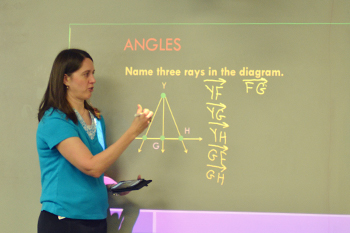Email a Teacher Prospective Families Optional Uniforms Apple Offering PowerSchool District: GTQF

Pre-Algebra: Teachers guide students through a full pre-algebra course and an introduction to geometry and discrete mathematics. Some topics covered include prime and composite numbers; fractions and decimals; order of operations, coordinates, exponents, square roots, ratios, algebraic phrases, probability, the Pythagorean theorem, and more.
Algebra 1: Students will develop the understanding they need to resolve more complex problems and functions: signed numbers, exponents; roots; absolute value; equations and inequalities; scientific notations; unit conversions; polynomials; graphs; factoring; quadratic equations; direct and inverse variations; exponential growth; statistics; and probability.
Geometry: Students learn triangle congruence, postulates and theorems, surface area and volume, two-column proofs, vector addition, and slopes and equations of lines.
Algebra 2: Teachers cover topics such as geometric functions, angles, perimeters, and proportional segments; negative exponents; quadratic equations; metric conversions; logarithms; and advanced factoring. Students also benefit from a thorough review of geometric principle.
Advanced Math: Teachers take students through an in-depth coverage of trigonometry, logarithms, analytic geometry, and upper-level algebraic concepts. Students benefit from continued practice of intermediate algebraic concepts and trigonometry introduced in Algebra 2 and learn new material such as functions, matrices, statistics, and using the graphing calculator.
Calculus (beginning 2019): Teachers equip future mathematicians, scientists, and engineers to build and reinforce their knowledge through continual practice and review. Following a condensed summary of key algebra, trigonometry, and analytic geometry topics, students explore limits, functions, and the differentiation and integration of variables.
Consumer Math: Teachers cover concepts such as buying, insuring, and maintaining a car; social security tax; creating and balancing a budget; renting a home; buying food and clothing; tax forms; and affording leisure time. Students learn sound, biblical views for managing money.

Not all advanced math electives are offered every year. Course availability is determined by factors such as enrollment and student demand.